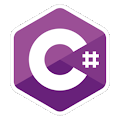Programming Course in C# ¡Free!

# Function Double

Proposed exercise

Create a function named "Double" to calculate and return an integer number doubled. For example. Double(7) should return 14.

Output

Solution

```using System;
public class FDouble
{
public static int Double(int number)
{
return number + number;
}

public static void Main()
{
Console.Write("Enter a number: ");